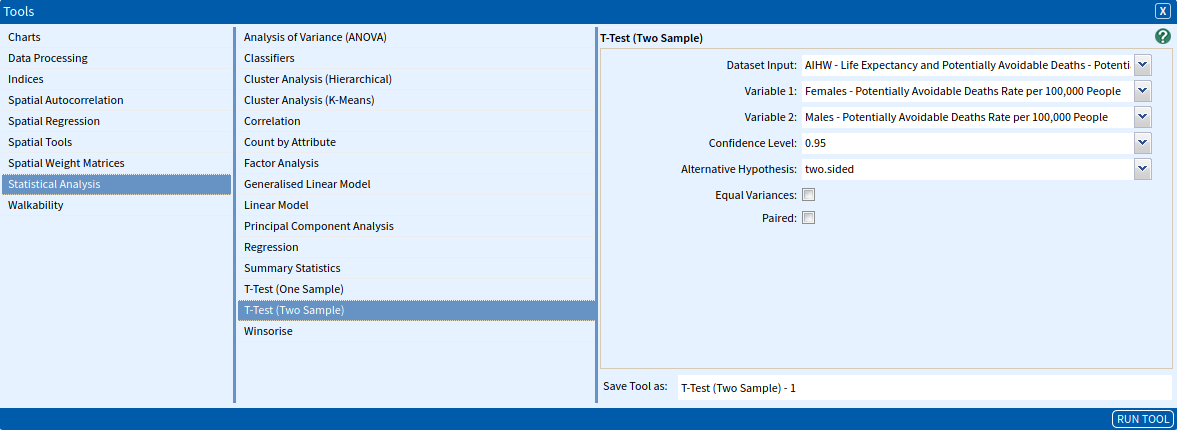# T-Test (Two Sample)

The T-test (Two Sample) tool is used to determine if the sample mean of a dataset is significantly different than a different dataset’s sample mean. It is commonly applied when the test statistic would follow a normal distribution if the value of a scaling term in the test statistic were known. When the scaling term is unknown and is replaced by an estimate based on the data, the test statistic (under certain conditions) follows a Student’s t distribution.

For example, it can be used to determine if there are significant differences between the rate of incidence of a disease between male and females, or if the mode of travel to work by train is significantly different to the use of motor vehicles.

### SET UP

For this worked example, we will compare the rates of potentially avoidable deaths per 100,000 people between males and females between 2014-2016 in Greater Brisbane.

Select the Greater Brisbane GCCSA as your area.

Select AIHW – Life Expectancy and Potentially Avoidable Deaths – Potentially Avoidable Deaths (%) (SA3) 2009-2016 as your dataset, with the following variables:

• SA3 Code
• SA3 Name
• Reporting Period: 2014-2016
• Females – Potentially Avoidable Deaths Rate per 100,000 People
• Males – Potentially Avoidable Deaths Rate per 100,000 People

### Inputs

Once you have set up your data, open the T-test (Two Sample) tool (Tools → Statistical Analysis → T-test (Two Sample)). The input fields are as follows:

• Dataset Input: The dataset containing the variables you would like to test. Select AIHW – Life Expectancy and Potentially Avoidable Deaths – Potentially Avoidable Deaths (%) (SA3) 2009-2016.
• Variable 1: The first variable we would like to test. Select Females – Potentially Avoidable Deaths Rate per 100,000 People.
• Variable 2: The variable we would like to test against the first variable. Select Males – Potentially Avoidable Deaths Rate per 100,000 People.
• Confidence Level: The confidence intervals for the calculation of means that will be undertaken as part of the test, ranging from 0.90 to 0.99. Select 0.95.
• Alternative Hypothesis: Specifies the alternative hypothesis. Select two.sided.
• two.sided: a priori assumption that the difference between the sample mean and the population mean is not equal to zero.
• greater: a priori assumption that the sample mean is greater than the population mean.
• less: a priori assumption that the sample mean is less than the population mean.
• Equal Variances: Indicates whether to treat the two variances as being equal. If TRUE then the pooled variance is used to estimate variance otherwise the Welch (or Satterthwaite) approximation to the degrees of freedom is used. Uncheck the box.
• Paired: Indicates whether to run a normal t-test or a paired t-test. A normal t-test should be selected if two independent samples are compared. In the example, our samples are independent since the rate of potentially preventable deaths for females and males are calculated using the female and male population of the SA3s respectively. Alternatively, if the rates were calculated using the total population of the SA3s, the samples would not be independent. Uncheck the box.

The input parameters are summarised in the image below, once complete click Run Tool.### Outputs

Once the tool has run, click the Display button on the pop-up dialogue box that appears. This will open a window with the outputs of your T-test (Two Sample), which should look something like the image below. The statistical significance is determined by the p-value. In this example, the p-value suggests that the rates of potentially preventable deaths between males and females are significantly different since the p-value is lower than 0.05.

The output values are as follows:

• t: The value of the t-statistic.
• df: The degrees of freedom for the t-statistic.
• p.value: The probability value for the t-statistic.
• Confidence level: The confidence level that you selected for your test.
• Confidence Interval: The confidence interval for the mean appropriate to the specified alternative hypothesis:
• two.sided: The confidence intervals include lower and upper limits.
• greater: The confidence intervals include lower limit and positive infinite.
• less: The confidence intervals include negative infinite and upper limit.
• mean of x: The mean of the Variable 1 values.
• mean of y: The mean of the Variable 2 values.
• method: The type of t-test.### Looking for Spatial Data?

You can browse the AURIN Data Discovery:### How can you Create Impact?